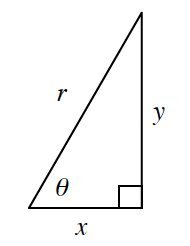### Home > PC3 > Chapter 2 > Lesson 2.2.2 > Problem2-65

2-65.In problem 2-58 you determined that  $\tan(θ)=\frac{y}{x}=\frac { \operatorname { sin } ( \theta ) } { \operatorname { cos } ( \theta ) }$ by using a unit circle. But what if the circle is not a unit circle? Consider the triangle in the diagram at right.

1. In the diagram, does $\tan(θ)=\frac{y}{x}$? Explain.

$\operatorname {tan} (\theta ) = \frac { \text { opposite } } { \text { adjacent } }$

2. Use the diagram to write ratios for $\cos(θ)$ and $\sin(θ)$

$\operatorname {cos} (\theta ) = \frac { \text { adjacent } } { \text { hypotenuse } }$

$\operatorname {sin} (\theta ) = \frac { \text { opposite } } { \text { hypotenuse } }$

3. Use your ratios from part (b) to write and simplify an expression $\frac { \operatorname { sin } ( \theta ) } { \operatorname { cos } ( \theta ) }$ that involves $x$, $y$, and $r$

$\frac{y/r}{x/r}$

4. Does $\tan(θ)=\frac{\sin(\theta)}{\cos(\theta)}$ for any right triangle? Explain. ​